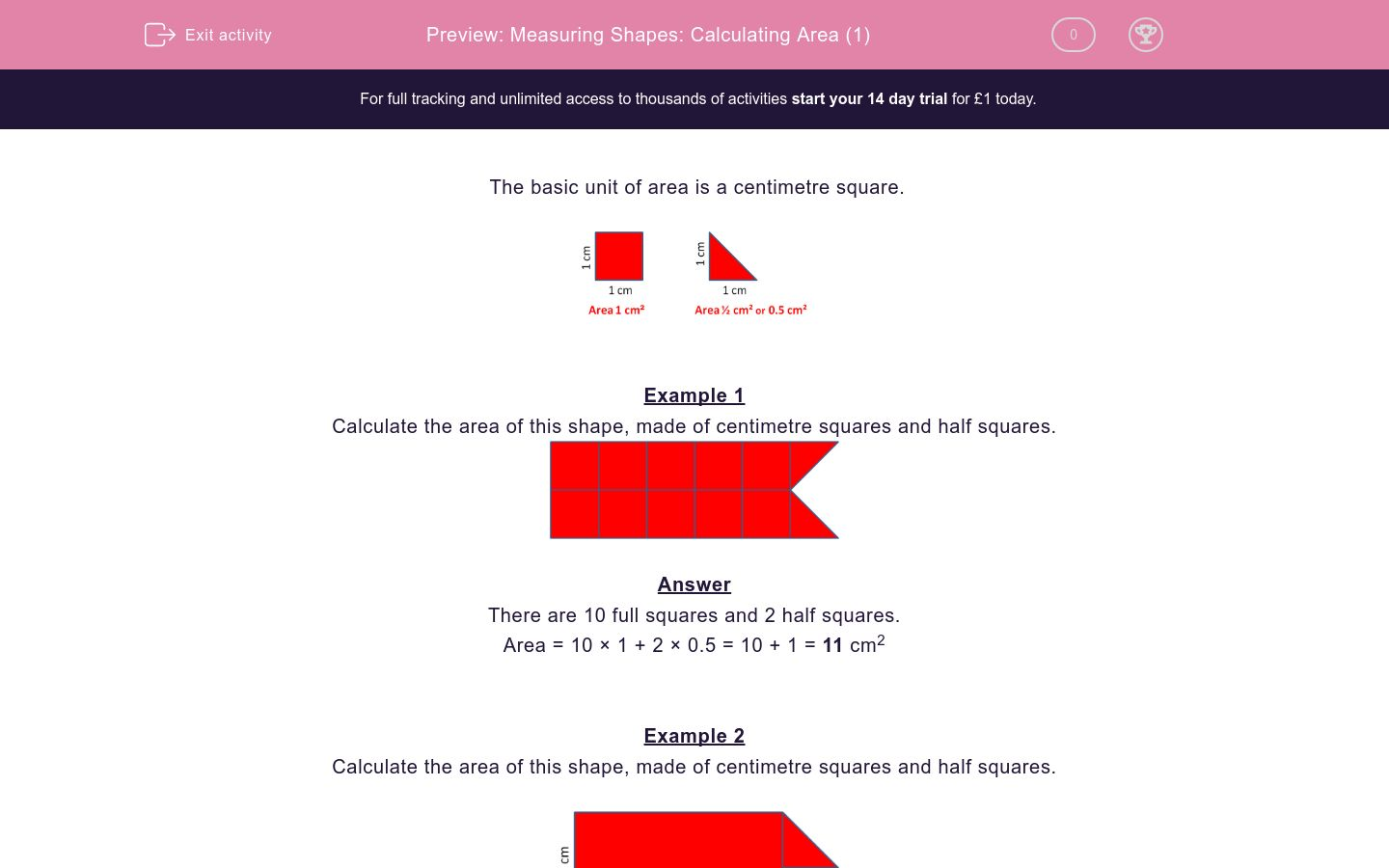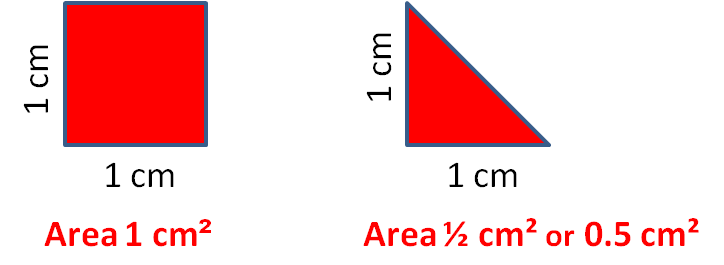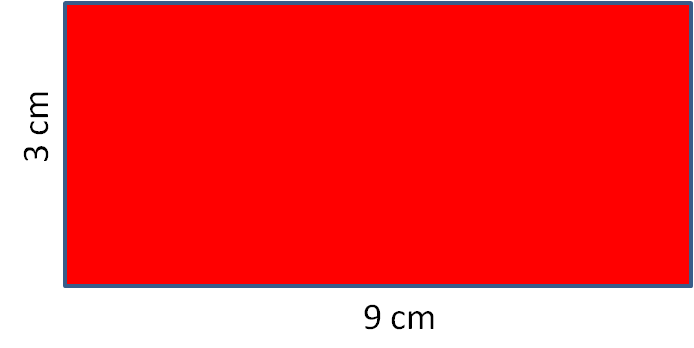# Measuring Shapes: Calculating Area (1)

In this worksheet, students work out the areas of simple shapes made of squares and half squares.Key stage:  KS 2

Curriculum topic:  Maths and Numerical Reasoning

Curriculum subtopic:  Perimeter and Area

Difficulty level:### QUESTION 1 of 10

The basic unit of area is a centimetre square.Example 1

Calculate the area of this shape, made of centimetre squares and half squares.There are 10 full squares and 2 half squares.

Area = 10 × 1 + 2 × 0.5 = 10 + 1 = 11 cm2

Example 2

Calculate the area of this shape, made of centimetre squares and half squares.The area of a rectangle = length × width

Area of rectangle = 3 × 2 = 6 cm2

Area of triangle = 0.5 cm2

Area of shape = 6 + 0.5 = 6.5 cm2

﻿Calculate the area of this shape, made of centimetre squares and half squares.

(Give your answer in cm2 and just write the number)﻿Calculate the area of this shape, made of centimetre squares and half squares.

(Give your answer in cm2 and just write the number)﻿Calculate the area of this shape, made of centimetre squares and half squares.

(Give your answer in cm2 and just write the number)﻿Calculate the area of this shape, made of centimetre squares and half squares.

(Give your answer in cm2 and just write the number)﻿Calculate the area of this shape, made of centimetre squares and half squares.

(Give your answer in cm2 and just write the number)﻿Calculate the area of this shape, made of centimetre squares and half squares.

(Give your answer in cm2 and just write the number)﻿Calculate the area of this shape, made of centimetre squares and half squares.

(Give your answer in cm2 and just write the number)﻿Calculate the area of this shape, made of centimetre squares.

(Give your answer in cm2 and just write the number)﻿Calculate the area of this shape, made of centimetre squares.

(Give your answer in cm2 and just write the number)﻿Calculate the area of this shape, made of centimetre squares and half squares.

(Give your answer in cm2 and just write the number)• Question 1

﻿Calculate the area of this shape, made of centimetre squares and half squares.

(Give your answer in cm2 and just write the number)7
• Question 2

﻿Calculate the area of this shape, made of centimetre squares and half squares.

(Give your answer in cm2 and just write the number)8
• Question 3

﻿Calculate the area of this shape, made of centimetre squares and half squares.

(Give your answer in cm2 and just write the number)6.5
• Question 4

﻿Calculate the area of this shape, made of centimetre squares and half squares.

(Give your answer in cm2 and just write the number)7.5
• Question 5

﻿Calculate the area of this shape, made of centimetre squares and half squares.

(Give your answer in cm2 and just write the number)8.5
• Question 6

﻿Calculate the area of this shape, made of centimetre squares and half squares.

(Give your answer in cm2 and just write the number)10
• Question 7

﻿Calculate the area of this shape, made of centimetre squares and half squares.

(Give your answer in cm2 and just write the number)8.5
• Question 8

﻿Calculate the area of this shape, made of centimetre squares.

(Give your answer in cm2 and just write the number)27
• Question 9

﻿Calculate the area of this shape, made of centimetre squares.

(Give your answer in cm2 and just write the number)35
• Question 10

﻿Calculate the area of this shape, made of centimetre squares and half squares.

(Give your answer in cm2 and just write the number)9
---- OR ----

Sign up for a £1 trial so you can track and measure your child's progress on this activity.

### What is EdPlace?

We're your National Curriculum aligned online education content provider helping each child succeed in English, maths and science from year 1 to GCSE. With an EdPlace account you’ll be able to track and measure progress, helping each child achieve their best. We build confidence and attainment by personalising each child’s learning at a level that suits them.

Get startedStart your £1 trial today.
Subscribe from £10/month.